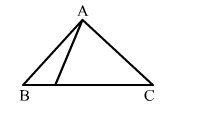# In the given figure, AB > AC. Then which of the following is true?

Question:

In the given figure, AB > AC. Then which of the following is true?
(d) Cannot be determinedSolution:

$A B>A C$ is given.

$\therefore \angle A C B>\angle A B C$

Now, $\angle A D B>\angle A C D \quad$ (exterior angle)

$\Rightarrow \angle A D B>\angle A C B>\angle A B C$

$\Rightarrow \angle A D B>\angle A B D \Rightarrow A B>A D$